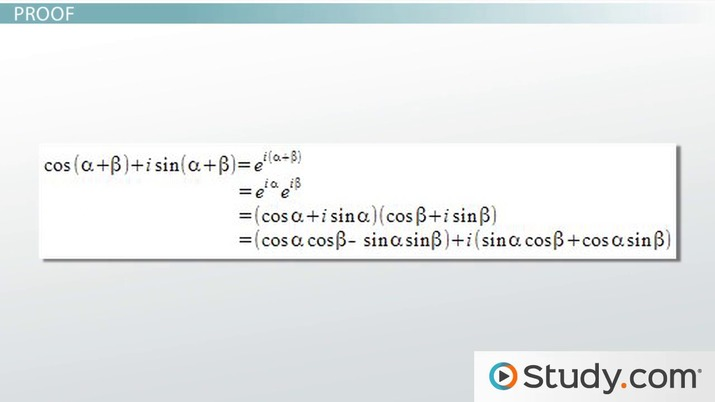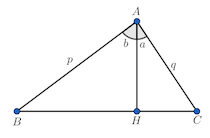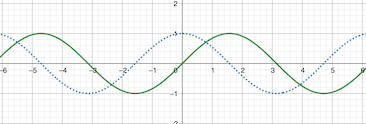Anderson Gomes Da Silva, Yuanxin (Amy) Yang Alcocer
• Author
Anderson Gomes Da Silva

Anderson holds a Bachelor's and Master's Degrees (both in Mathematics) from the Fluminense Federal University and the Pontifical Catholic University of Rio de Janeiro, respectively. He was a Teaching Assistant at the University of Delaware (UD) for two and a half years, leading discussion and laboratory sessions of Calculus I, II and III. In the Winter of 2021 he was the sole instructor for one of the Calculus I sections at UD.

• Instructor
Yuanxin (Amy) Yang Alcocer

Amy has a master's degree in secondary education and has been teaching math for over 9 years. Amy has worked with students at all levels from those with special needs to those that are gifted.

Show

## Trig Addition and Subtraction Identities

The three main trigonometric functions are sine, cosine, and tangent and, to learn them, the unit circle is used, which is centered at the origin {eq}(0, 0) {/eq} with radius {eq}1. {/eq} There are usually multiple ways of expressing information that involves trigonometric functions. When these equivalent expressions are written as equalities, we have trigonometric identities. For example, if there is an angle {eq}c {/eq} that is the sum of two other angles, say, {eq}a {/eq} and {eq}b, {/eq} it can be expressed, for example, {eq}\sin (c), \cos (c), \tan (c) {/eq} in terms of {eq}\sin (a), \sin (b), \cos (a), \cos (b), \tan (a), \tan (b). {/eq} When this is done, it is known as trig addition identities and that is the focus of this lesson.

Angles {eq}30^{\circ}, 40^{\circ} {/eq} and {eq}60^{\circ} {/eq} are important, their trigonometric ratios can be easily determined and such values are even memorized. But that is not the case for other angles such as {eq}75^{\circ} = 30^{\circ} + 45^{\circ} {/eq} or {eq}15^{\circ} = 60^{\circ} - 45^{\circ}. {/eq} Therefore, the identities derived here will determine the trig ratios of other angles, like those mentioned above. Another reason why trig addition identities are important is that they were helpful when calculators were not here.An error occurred trying to load this video.

Try refreshing the page, or contact customer support.

Coming up next: List of the Basic Trig Identities

### You're on a roll. Keep up the good work!

Replay
Your next lesson will play in 10 seconds
• 1:28 Proof
• 3:33 Uses
• 4:27 Example
• 5:35 Lesson Summary
Save Save

Want to watch this again later?

Timeline
Autoplay
Autoplay
Speed Speed

## Trig Addition and Subtraction Formulas

As we saw in the previous section, trig addition identities are relevant. Here we are going to see how to represent the sine, cosine, and tangent of the sum or difference of two angles, that is, we are going to present addition and subtraction formulas.

### Sine Addition Formula and Sine Subtraction Formula

Let {eq}a {/eq} and {eq}b {/eq} be two angles. Then, sine addition formula and sine subtraction formula are given by

$$\sin (a + b) = \sin (a) \cos (b) + \sin (b) \cos (a) ~ \textrm{and} ~ \sin (a - b) = \sin (a) \cos (b) - \sin (b) \cos (a)$$

For simplicity, it can be written as $$\sin (a \pm b) = \sin (a) \cos (b) \pm \sin (b) \cos (a)$$

### Cos Addition Formula and Cos Subtraction Formula

Subtraction can be perceived as an addition with a negative term. So, it can be said that the cos addition formula is given by $$\cos (a \pm b) = \cos (a) \cos (b) \mp \sin (a) \sin (b)$$

### Tangent Addition Formula and Subtraction Formula

Tangent addition formula is given by

$$\tan (a \pm b) = \frac{\tan (a) \pm \tan (b)}{1 \mp \tan (a) \tan (b)}$$

The reasoning for {eq}\pm {/eq} and {eq}\mp {/eq} is similar to the one used for sine and cosine.

## Proof for Trig Addition Formulas

First were the trig addition formulas and now it is their proofs. Let {eq}ABC {/eq} be a triangle with {eq}AH {/eq} being a height of the triangle. The segment {eq}AH {/eq} divides the angle {eq}\angle BAC {/eq} in two angles {eq}\angle BAH = b {/eq} and {eq}\angle HAC = a, {/eq} as in the following picture.The sum of the two smaller triangles equals the area of the biggest one. This information is going to be used, along with {eq}\cos a = \frac{h}{q} {/eq} and {eq}\cos b = \frac{h}{p}. {/eq} We have

• {eq}A_{ABC} = \frac{pq}{2}\sin (a + b) {/eq}
• {eq}A_{ABH} = \frac{ph}{2}\sin (b) {/eq}
• {eq}A_{ACH} = \frac{hq}{2}\sin (a) {/eq}

{eq}A_{ABC} = A_{ABH} + A_{ACH} \implies \frac{pq}{2} \sin (a + b) = \frac{ph}{2}\sin (b) + \frac{hq}{2}\sin (a) \implies \sin (a + b) = \frac{h}{q}\sin (b) + \frac{h}{p}\sin (a). {/eq} Plugging {eq}\cos (a) {/eq} and {eq}\cos (b) {/eq} in the equation above gives {eq}\sin (a + b) = \sin (a) \cos (b) + \sin (b) \cos (a). {/eq}

To prove the cosine sum formula, consider the following image, which shows that the graph of {eq}\cos x {/eq} can be obtained from the graph of {eq}\sin x {/eq} by shifting it {eq}\frac{\pi}{2} {/eq} to the left.Algebraically, {eq}\sin (x + \pi/2) = \cos (x) {/eq} and {eq}\cos (x) = \sin (x + \pi/2) {/eq}

To unlock this lesson you must be a Study.com Member.

#### What is the sine addition formula?

Consider angles a and b whose measures are given in radians. Then, sin (a + b) = sin (a) x cos (b) + sin (b) x cos (a).

#### What is the addition formula for cos?

Let a and b be angles given in radians. Then, cos (a + b) = cos (a) x cos (b) - sin (a) x sin (b)

### Register to view this lesson

Are you a student or a teacher?